# Excel-VBA基础语法（VBA简介、数据类型、变量、数组、运算符、内置函数、过程与函数）2021-10-06 21:25:11   来源：   评论：0 点击：

1、宏和VBA的关系

vba是编程语言，宏是用vba代码保存下来的程序。录制的宏是vba里最简单的程序，正因为如此，录制宏存在许多缺陷：如无法进行判断和循环，不能显示用户窗体，不能进行人机交互……

解决录制宏的这些问题，需要掌握vbs编程的方法，自主的编写vba程序。

2、VBA程序结构

• 代码：vba程序由代码组成。
• 过程：例如Sub过程、Function过程
• 模块：保存过程的地方，一个模块可以保存多个不同类型的过程
• 对象：用代码操作或控制的东西即为对象，例如工作簿、工作表、单元格、图片、图表、透视表等
• 对象的属性：每个对象都有属性，属性是对象包含的内容或特点，例如A1单元格的内容：A1.内容，代码表达为Range("A1).Value
• 对象的方法：方法是指在对象上执行的某个动作，例如Range("A1").Select
• 关键字：关键字是vba中的保留字或符号，例如语句名称、函数名称、运算符等都是关键字

3、VBE介绍

VBE即Visual Basic Editor即VBA的编程环境

1）打开VBE编辑器

Alt+F11（Alt+F8是查看宏）

依次执行：（2003版本）工具——宏——Visual Basic编辑器，（2007以上版本）在“视图”下的“宏”选项卡下

右键单击工作表标签，执行“查看代码”命令

2）主窗口：包含“工程资源管理器”、“属性窗口”、“菜单栏”、“工具栏”、“代码窗口”、“立即窗口”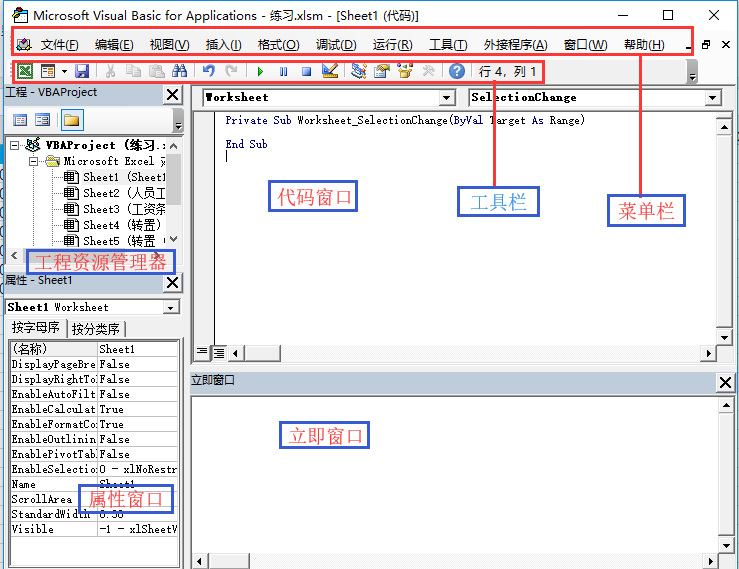3）菜单栏：包含VBE中各种组件的命令

4）工具栏：可以在“视图”——“工具栏”菜单里显示或隐藏

5）工程资源管理器：在这里可以看到所有打开的Excel工作簿和已加载的宏，一个Excel的工作簿就是一个工程，工程名称为“VBA Project（工作簿名称）”，这里最多可以显示工程里的4类对象，即Excel对象（包括sheet对象和ThisWorkbook对象）、窗体对象、模块对象和类模块对象。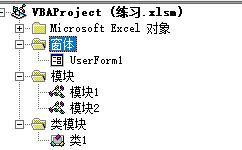6）属性窗口：在这里查看或设置对象的属性

7）代码窗口：包含对象列表框、过程列表框、边界标识条、视图按钮、代码编辑区、过程分界线。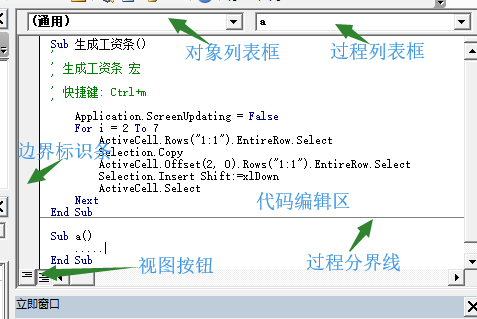8）立即窗口：一个重要用途是用来调试代码，想显示立即窗口，可以在视图选项卡中选择或者用快捷键“Ctrl+G”

4、牛刀小试：用vba生成工资条

```Sub 生成工资条()
'
' 生成工资条 宏
'
' 快捷键: Ctrl+m
'
Application.ScreenUpdating = False
For i = 2 To 7
ActiveCell.Rows("1:1").EntireRow.Select
Selection.Copy
ActiveCell.Offset(2, 0).Rows("1:1").EntireRow.Select
Selection.Insert Shift:=xlDown
ActiveCell.Select
Next
End Sub```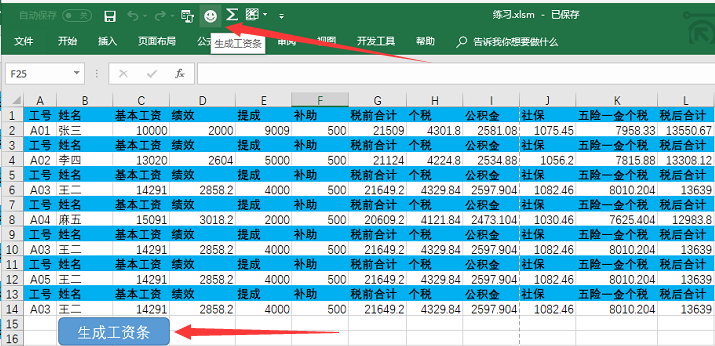1、VBA中的数据类型

VBA里的数据类型有：字节型（Byte）、整数型（Integer）、长整数型（Long）、单精度浮点型（Single）、双精度浮点型（Double）、货币型（Currency）、小数型（Decimal）、字符串型（String）、日期型（Date）、布尔型（Boolean）等，如表3-1

类型声明符：用特殊符号代替变量类型进行变量类型声明，例如Dim str\$   中\$代表String类型。只有部分数据类型可以使用类型声明符。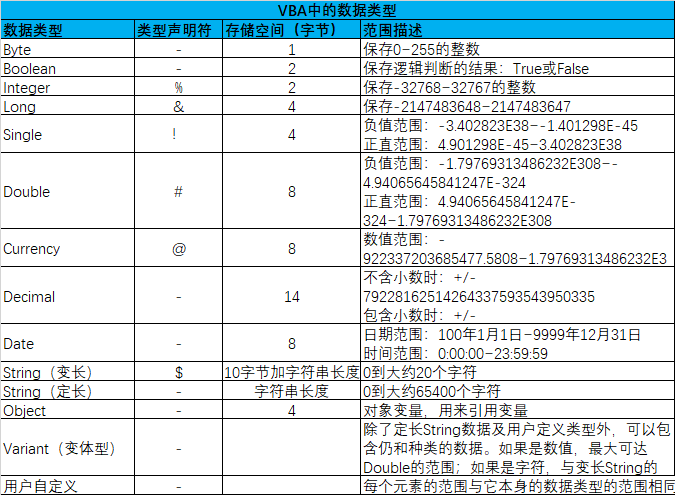1、变量命名要求

2、声明变量

• 单变量定义：Dim 变量名 As 数据类型

示例：

Dim str As String　　声明一个String类型（变长）的变量，名称是str

Dim str As String*10　　声明一个String类型（定长，最大存储10个字符）的变量，名称为str

Dim str\$　　声明一个String（变长）类型变量，\$变量类型声明符，代表String

• 多变量定义（变量类型相同）：Dim 变量1,变量2,…… As 数据类型

Dim x, y, z As String

• 多变量定义（变量类型不同）：Dim 变量1 As 数据类型1,变量2 As 数据类型2

Dim str As String,nu As Integer　　不同变量之间用逗号隔开

• 　　不指定类型的变量定义，默认为Variant类型

Dim str　　每个变量都要指定数据类型，如果不指定，默认为Variant类型

3、声明常量

Const pi As Single=3.14

4、变量的作用域

Public 变量名称  As 数据类型　　【公有变量】

Private 变量名称  As 数据类型 　　【私有变量】

Static 变量名称 As 数据类型　　【静态变量，整个代码运行期间值不变】

5、强制声明所有变量（未定义变量则提示，否则不提示）

Option Explicit　　在模块的第一句表示。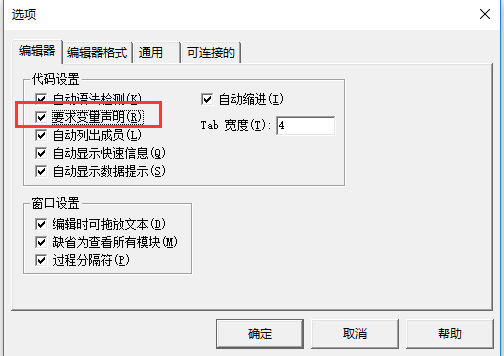6、给变量赋值

• 给文本、数值、日期等数据类型变量赋值

语句为：[Let]变量名称 = 数据　　这里的Let可以省略，即：变量名称=数据

例如：

Dim str As String

Let str = “一起来学习VBA”

• 给对象变量（object型，例如单元格）赋值

语句为：Set 变量名称=对象　　这里Set千万不能少。

例如：

Dim rng = Range　　　　'声明rng变量为Range类型

Set rng = Worksheets("sheet1").Range("A1")　　'给变量rng赋值

rng.Value="欢迎来到ExcelHome论坛"　　　　'将文本写入变量指定的单元格

1、一维数组

Dim 七6班(1 to 50) As String  '声明一个String类型的数组，名称为“七6班”，可以存储50个元素。

Public|Dim Arr(0 to 49) As String

Public|Dim Arr(49) As String   '数组索引默认是从0开始计数，如果在模块的第一句写“Option Base 1”，则数组的起始索引从1开始，而不是0.

七6班(1)="张青"

七6班(2)="邓城"

……

七6班(50)="冯吉"

2、多维数组：

Dim 酱油(1 to 3,1 to 20)

Dim 酱油(2,19)

3、多维动态数组

不确定数组中存储多少个元素，即不能预知元素的大小，可以在首次定义数组时括号内为空，写成：

Dim 数组名称()

```Sub dtsz()
Dim arr() As String '定义多维动态数组
Dim n As Long
'统计A列有多少非空单元格
n = Application.WorksheetFunction.CountA(Range("A:A"))
MsgBox n
'使用Dim语句声明变量时，括号内的参数不能是变量，所以必须使用ReDim语句重新指定大小
ReDim arr(1 To n) As String '重新定义数组的大小
End Sub```

4、其他常用的创建数组的方式

1）使用Array函数创建数据

```Sub ArrayTest()
Dim arr As Variant '定义变量
'将1-10的自然数赋给数组
arr = Array(1, 2, 3, 4, 5, 6, 7, 8, 9, 10)
MsgBox "arr数组的第2个元素为：" & arr(1)
End Sub```

2）使用split创建数组

```Sub ArrayTest()
Dim arr As Variant '定义变量
'无论是否在模块中写入Option Base 1，Split函数返回的数组的最小索引都是0
arr = Split("邓成,林梅,张青,孔丽,冯吉维,孔佳", ",") '第一个参数包含分隔符或字符串变量，第二个参数是分隔符
MsgBox "arr数组的第2个元素为：" & arr(1)
End Sub```

3）通过Range对象直接创建数组

```Sub ArrayTest()
Dim arr As Variant '定义变量
arr = Range("A1:C3").Value '将A1:C3单元格内容存储到数组arr里
Range("E1:G3").Value = arr '将数组arr的数据写入大E1:G3
'将数组的值写入到单元格区域时，单元格区域的大小必须与数组相同
End Sub```

5、数组相关函数：UBound和LBound函数

UBound(arr) 获取数组arr的最大索引号

LBound(arr)  获取数组arr的最小索引号

```Sub arrcount()
Dim arr(10 To 50)
'Char(13)表示回车,_表示代码换行连接符
MsgBox "数组的最大索引号是：" & UBound(arr) & Chr(13) _
& "数组最小的索引号是：" & LBound(arr) & Chr(13) _
& "数组的元素个数是：" & UBound(arr) - LBound(arr) + 1
End Sub```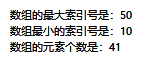```Sub arrcount()
Dim arr(1 To 10, 1 To 100)
'Char(13)表示回车,_表示代码换行连接符
MsgBox "第一维的最大索引号是：" & UBound(arr, 1) & Chr(13) _
& "第二维的最小索引号是：" & LBound(arr, 2)
End Sub```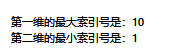6、数组相关函数：Join函数

```Sub joinTest()
Dim arr As Variant, txt As String
arr = Array(0, 1, 2, 3, 4, 5, 6, 7, 8, 9)
'分割符@可以省略，如果省略，默认使用空格作为分隔符
txt = Join(arr, "@")
MsgBox txt
End Sub```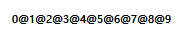```Sub ArrToRng1()
Dim arr As Variant
arr = Array(1, 2, 3, 4, 5, 6, 7, 8, 9)
'将一维数组写入单元格区域，单元格区域必须在同一行。如果要写入垂直的一列单元格区域，必须先使用工作表的Transpose函数进行转换
Range("A1:A9").Value = Application.WorksheetFunction.Transpose(arr)
End Sub```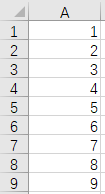1、算术运算符

用于算术运算，返回值类型为数值型。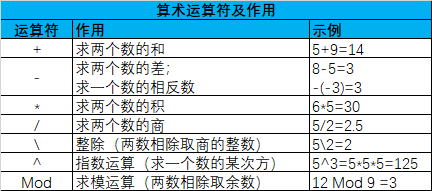2、比较运算符

用于比较运算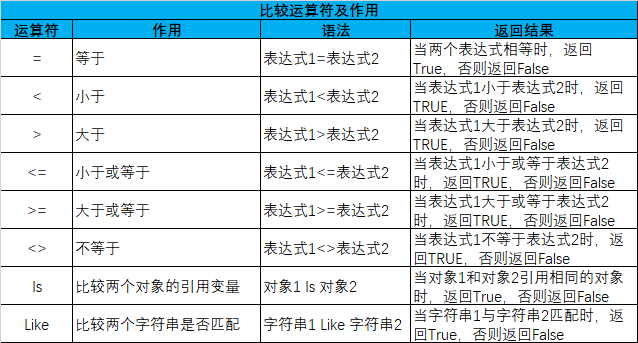3、连接运算符

连接运算符用来连接两个文本字符串，有+和&两种

a="欢迎来到"

b="ExcelHome论坛！"

?a+b 　　　　　　'问号?告诉VBA在立即窗口中显示问号后面命令的结果，可以用Print关键字代替问号。

?a & b

?4+5 '符号+两边都是数值，执行算术运算

9

?"4"+5 '其中5是数值，执行算术运算

9

?"4"+"5" '两个都是文本，执行连接运算

45

4、逻辑运算符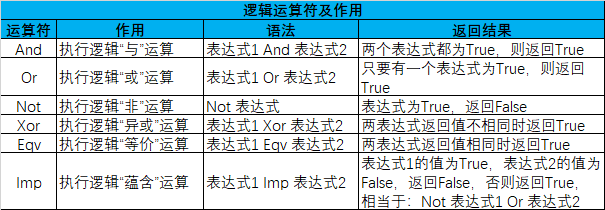5、VBA中的通配符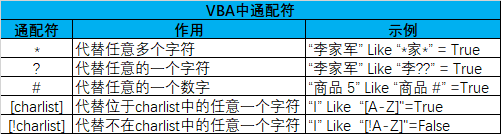6、运算符优先级

在VBA中要优先处理蒜素运算符，接着处理连接运算符，然后处理比较运算符，最后再处理逻辑运算符，可以用括号来改变运算顺序。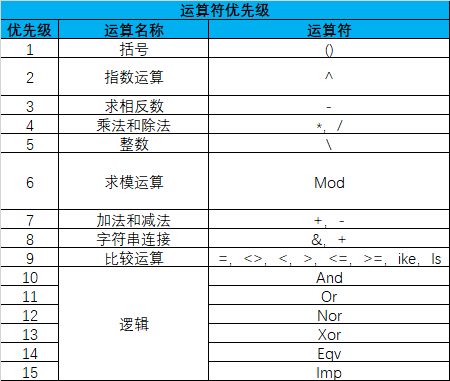7、换行符

```VBA中字符换行显示需要使用换行符来完成。下面是常用的换行符
'chr(10) 可以生成换行符
'chr(13) 可以生成回车符
'vbcrlf 换行符和回车符
'vbCr 等同于chr(10)
'vblf 等同于chr(13)
'例：
Sub test3()
MsgBox "我爱" & Chr(10) & "Excel"
' MsgBox "我爱你" & Chr(13) & "Excel"
' MsgBox "今天" & vbCrLf & "我是大王"

End Sub```

```Sub NowTime()
MsgBox "现在的时间是：" & Time()
End Sub```

VBA中的内置函数有哪些？查看VBA内置函数的方法：

1、在VBE中“帮助（H）”——“Microsoft Visual Basic 帮助（H） F1”——“Visual Basic 语言参考”——“函数” 或者在VBE下快捷键“F1”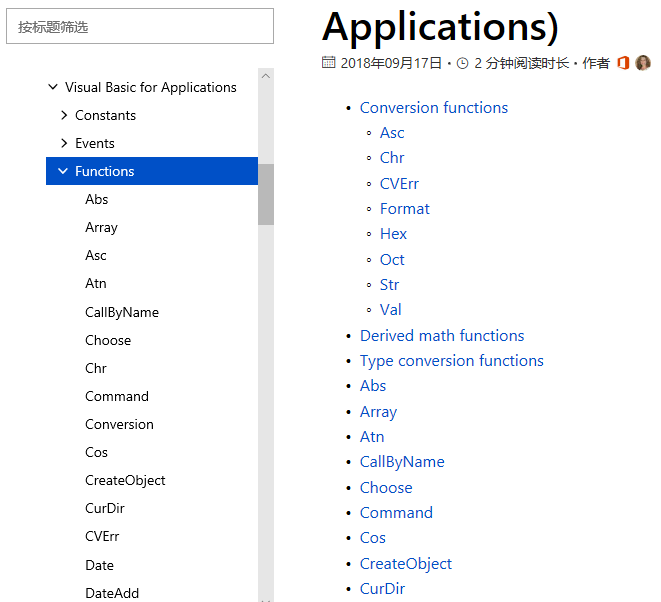2、在VBE代码窗口中首先键入“VBA.”系统会自动提示“函数列表”，如图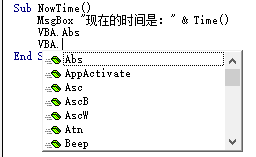1、If…Then语句

```Sub SayHello1()
If Time < 0.5 Then MsgBox "早上好!"
If Time >= 0.5 Then MsgBox "下午好！"
End Sub

Sub SayHello2()
If Time < 0.5 Then
MsgBox "早上好!"
Else
MsgBox "下午好！"
End If
End Sub

Sub SayHello3()
If Time < 0.5 Then
MsgBox "早上好!"
ElseIf Time > 0.75 Then
MsgBox "晚上好！"
Else
MsgBox "下午好！"
End If
End Sub```

2、Select Case语句

```Sub SayHello1()
Select Case Time
Case Is < 0.5
MsgBox "早上好!"
Case Is > 0.75
MsgBox "晚上！"
End Select
End Sub

Sub SayHello2()
Select Case Time
Case Is < 0.5
MsgBox "早上好!"
Case Is > 0.75
MsgBox "晚上！"
Case Else
MsgBox "下午好！"
End Select
End Sub```

```Sub xingji()
Dim xj As String
Select Case Cells(2, "H")
Case Is < 85
xj = "不评定"
Case Is < 100
xj = "一星级"
Case Is < 115
xj = "二星级"
Case Is < 130
xj = "三星级"
Case Is < 150
xj = "四星级"
Case Else
xj = "五星级"
End Select
Cells(2, "I") = xj
End Sub```

3、For…Next语句

语法结构：

```For <循环变量>=<初值>To<终值> [Step 步长值]
<循环体>
[Exit For]  '可以在循环体中任意处加一句或多句Exit For，当遇到这个语句，退出For循环，执行Next后语句
<循环体>
Next [循环变量]```

```Sub xingji()
Dim xj As String, i As Integer
For i = 2 To 19 Step 1
Select Case Cells(i, "H")
Case Is < 85
xj = "不评定"
Case Is < 100
xj = "一星级"
Case Is < 115
xj = "二星级"
Case Is < 130
xj = "三星级"
Case Is < 150
xj = "四星级"
Case Else
xj = "五星级"
End Select
Cells(i, "I") = xj
Next i
End Sub```

4、Do While语句

• 语法结构1：
```Do [While 逻辑表达式]
<循环体>
[Exit Do]
[循环体]
Loop```

```Sub xingji()
Dim xj As String, i As Integer
i = 2
Do While Cells(i, "H") <> ""
Select Case Cells(i, "H")
Case Is < 85
xj = "不评定"
Case Is < 100
xj = "一星级"
Case Is < 115
xj = "二星级"
Case Is < 130
xj = "三星级"
Case Is < 150
xj = "四星级"
Case Else
xj = "五星级"
End Select
Cells(i, "I") = xj
i = i + 1
Loop
End Sub```
• 语法结构2
```Do
<循环体>
[Exit Do]
[循环体]
Loop  [While 逻辑表达式]```

```Sub xingji()
Dim xj As String, i As Integer
i = 2
Do
Select Case Cells(i, "H")
Case Is < 85
xj = "不评定"
Case Is < 100
xj = "一星级"
Case Is < 115
xj = "二星级"
Case Is < 130
xj = "三星级"
Case Is < 150
xj = "四星级"
Case Else
xj = "五星级"
End Select
Cells(i, "I") = xj
i = i + 1
Loop While Cells(i, "H") <> ""
End Sub```

5、Do Until语句

do Until 后的逻辑表达式为False则执行循环体，否则退出循环，跟Do While相反。

• 语法结构1：
```Do [Until 逻辑表达式]
<循环体>
[Exit Do]
[循环体]
Loop```

对应示例：

```Sub xingji()
Dim xj As String, i As Integer
i = 2
Do Until Cells(i, "H") = ""
Select Case Cells(i, "H")
Case Is < 85
xj = "不评定"
Case Is < 100
xj = "一星级"
Case Is < 115
xj = "二星级"
Case Is < 130
xj = "三星级"
Case Is < 150
xj = "四星级"
Case Else
xj = "五星级"
End Select
Cells(i, "I") = xj
i = i + 1
Loop
End Sub```
• 语法结构2：
```Do
<循环体>
[Exit Do]
[循环体]
Loop [Until 逻辑表达式]```

对应示例：

```Sub xingji()
Dim xj As String, i As Integer
i = 2
Do
Select Case Cells(i, "H")
Case Is < 85
xj = "不评定"
Case Is < 100
xj = "一星级"
Case Is < 115
xj = "二星级"
Case Is < 130
xj = "三星级"
Case Is < 150
xj = "四星级"
Case Else
xj = "五星级"
End Select
Cells(i, "I") = xj
i = i + 1
Loop Until Cells(i, "H") = ""
End Sub```

6、For Each…Next语句

```For Each 元素变量 In 集合名称或数组名称
<语句块1>
[Exit For]
[语句块2]
Next [元素变量]```

```Sub shtName()
Dim sht As Worksheet, i As Integer
i = 1
For Each sht In Worksheets
Cells(i, "A") = sht.Name
i = i + 1
Next sht
End Sub```

```Sub shtName()
Dim c As Range, i As Integer
i = 1
For Each c In Range("A1:A10")
c.Value = i
i = i + 1
Next c
End Sub```

7、GoTo语句

“去到指定地点”，用来让程序转到另外一条语句去执行。

```Sub he()
Dim mysum As Long, i As String
i = 1
x:    mysum = mysum + i
i = i + 1
If i <= 100 Then GoTo x
MsgBox "1到100的自然数和是：" & mysun

End Sub```

8、With语句

当需要对相同的对象进行多次操作时，会编写一些重复代码

```Sub FontSet()
With Worksheets("sheet1").Range("A1").Font
.Name = "仿宋"
.Size = 12
.Bold = True
.ColorIndex = 3
End With  '这里的With语句结束标志，不可缺少
End Sub ```

1、Sub定义语句

```'所有[]内容都是可选的
'Exit Sub：可选语句，执行它将中断执行并退出过程
'如果选用Static，运行程序的过程中将保存该过程里声明的本地变量
'Private和Public用于声明过程的作用域名，如果省略，过程默认为公共过程
[Private|Public][Static] Sub 过程名([参数列表])
[语句块]
[Exit Sub]
[语句块]
End Sub ```

2、Sub间调用

```Sub SayHello()
If Time < 0.5 Then
MsgBox "早上好!"
ElseIf Time > 0.75 Then
MsgBox "晚上好！"
Else
MsgBox "下午好！"
End If
End Sub```

```Sub RunSub()
SayHello
End Sub```

```Sub RunSub()
Call SayHello
End Sub```

```Sub RunSub()
Application.Run "SayHello"
End Sub```

3、过程的作用域

公共过程：可以跨模块调用，用Public 或省略不写。

```Public Sub gggc()
MsgBox "我是公共过程"
End Sub```

私有过程：只能在模块内调用，用Private

```Private Sub gggc()
MsgBox "我是私有过程"
End Sub```

Function过程也称为函数过程，编写一个Function过程，就是编写一个函数。

1、定义语法格式

```'最后必须将结果赋值给函数名称
[Private|Public][Static] Function 函数名([参数列表])[As 数据类型]
[语句块]
[函数名=过程结果]
[Exit Function]
[语句块]
[函数名=过程结果]
End Function```

2、定义函数

```Public Function Fun()
Fun = Int(Rnd() * 10) + 1
End Function```

3、使用函数

• 使用函数方法一：直接在单元格加“=”调用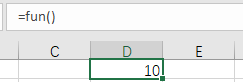• 使用函数方法二：通过“函数”——“用户定义” 选择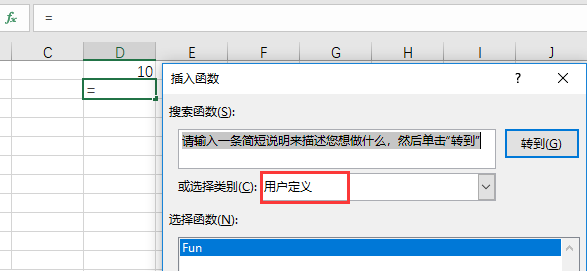• 用户定义函数可以和其他函数嵌套使用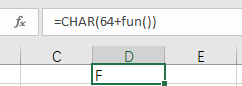•  在VBA过程中使用自定义函数
```Sub msg()
MsgBox Fun()
End Sub```

4、函数实例

RGB函数中，R代表红色，G代表绿色，B代表蓝色

RGB(255,255,0)  表示黄色

• 判断单元格是否是黄色，是则返回1，否则返回0
```'最后必须将结果赋值给函数名称
Function CountColor()
If Range("A1").Interior.Color = RGB(255, 255, 0) Then
CountColor = 1 '如果是黄色，函数值等于1
Else
CountColor = 0
End If
End Function```
• 统计指定颜色的单元格个数
```'最后必须将结果赋值给函数名称
Function CountColor()
Dim rng As Range
For Each rng In Range("A1:A10")
If rng.Interior.Color = RGB(255, 255, 0) Then
CountColor = CountColor + 1
End If
Next rng
End Function```
• 用参数指定计算区域
```'最后必须将结果赋值给函数名称
Function CountColor(arr As Range)
Dim rng As Range
For Each rng In arr
If rng.Interior.Color = RGB(255, 255, 0) Then
CountColor = CountColor + 1
End If
Next rng
End Function```
• 给自定义函数第2个参数
```'最后必须将结果赋值给函数名称
Function CountColor(arr As Range, c As Range)
Dim rng As Range
For Each rng In arr
If rng.Interior.Color = c.Interior.Color Then
CountColor = CountColor + 1
End If
Next rng
End Function```
• 设置自定义函数为易失性函数

```'最后必须将结果赋值给函数名称
Function CountColor(arr As Range, c As Range)
Application.Volatile True
Dim rng As Range
For Each rng In arr
If rng.Interior.Color = c.Interior.Color Then
CountColor = CountColor + 1
End If
Next rng
End Function ```

```Sub test()
Application.Workbooks("Book1").Worksheets("sheet1") _
.Range("A1:D100").Font.Bold = True
End Sub```

```Sub test()
Dim a%, b%, c%: a = 1: b = 2: c = 3
End Sub```

```Sub test()
'=================注释
Application.Workbooks("Book1").Worksheets("sheet1") _
.Range("A1:D100").Font.Bold = True
Rem ==============注释
End Sub```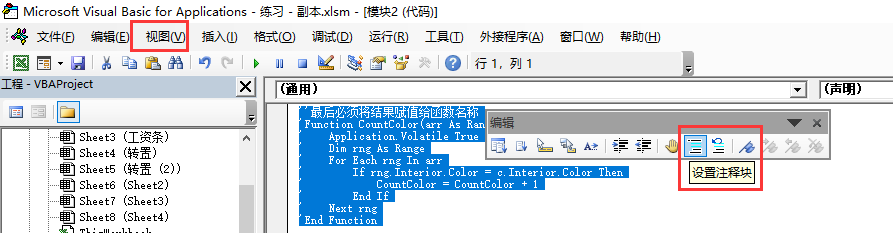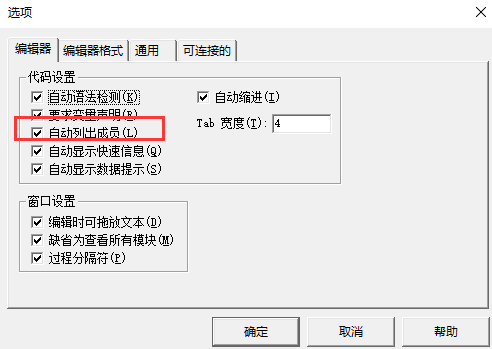https://www.cnblogs.com/wzh313/articles/9737573.html